Welcome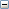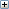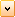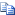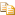Creates a trimming loop out of < CurveCount > surface parametric (UV-curves) and adds it to a list of such loops.

# .NET Syntax

Visual Basic (Declaration)
```Sub IAddTrimmingLoop2( _
ByVal CurveCount As System.Integer, _
ByRef Order As System.Integer, _
ByRef Dim As System.Integer, _
ByRef Periodic As System.Integer, _
ByRef NumKnots As System.Integer, _
ByRef NumCtrlPoints As System.Integer, _
ByRef Knots As System.Double, _
ByRef CtrlPointDbls As System.Double, _
ByRef UvRange As System.Double _
) ```
Visual Basic (Usage)
```Dim instance As ISurface
Dim CurveCount As System.Integer
Dim Order As System.Integer
Dim Dim As System.Integer
Dim Periodic As System.Integer
Dim NumKnots As System.Integer
Dim NumCtrlPoints As System.Integer
Dim Knots As System.Double
Dim CtrlPointDbls As System.Double
Dim UvRange As System.Double

instance.IAddTrimmingLoop2(CurveCount, Order, Dim, Periodic, NumKnots, NumCtrlPoints, Knots, CtrlPointDbls, UvRange)```
C#
```void IAddTrimmingLoop2(
System.int CurveCount,
ref System.int Order,
ref System.int Dim,
ref System.int Periodic,
ref System.int NumKnots,
ref System.int NumCtrlPoints,
ref System.double Knots,
ref System.double CtrlPointDbls,
ref System.double UvRange
)```
C++/CLI
```void IAddTrimmingLoop2(
&   System.int CurveCount,
&   System.int% Order,
&   System.int% Dim,
&   System.int% Periodic,
&   System.int% NumKnots,
&   System.int% NumCtrlPoints,
&   System.double% Knots,
&   System.double% CtrlPointDbls,
&   System.double% UvRange
) ```

#### Parameters

CurveCount

Number of surface parametric (UV) curves constituting the loop; it is also directly related (see Remarks) to the dimension of each of the arrays Order, Dim, Periodic, NumKnots, NumControlPnts, which must be loaded with the information regarding each of the curves; specifically the dimension of those arrays is ArraySize = CurveCount

Order
Array of <ArraySize> longs (see CurveCount argument)
Dim
Array of <ArraySize> longs (see CurveCount argument); if you set the first value in this array to negative its absolute value, then 3D trim curves are expected
Periodic
Array of <ArraySize> longs (see CurveCount argument)
NumKnots
Array of <ArraySize> longs (see CurveCount argument)
NumCtrlPoints
Array of <ArraySize> longs (see CurveCount argument)
Knots
Array of <TotalNumKnots> doubles, where TotalNumKnots = numKnotsInCurve[i] from i = 1 to CurveCount
CtrlPointDbls
Array of <TotalNumCPCoords> doubles, where TotalNumCPCoords = ( dimensionOfCPinCurve[i] * numControlPntsInCurve[i] ) from i = 1 to CurveCount
UvRange
Array of four doubles defining U Low U High V Low V High

# Remarks

The list is created by a previous call to one of the base surface creation functions (for example, IBody2::CreateRevolutionSurface or IBody2::ICreateRevolutionSurface).

The information on each UV-curve is in b-spline form (knots and control point coordinates) all of which are compacted into the two arrays Knots and CtrlPointDbls.

For non-rational curves the dimension value in Dim is 2. For rational curves the dimension value in Dim is 3. The minimum order value in Order is 2. An order 2 curve must consist of only one segment.

For each curve the number of control points in NumCtrlPoints >= its order.

For non-periodic curves there must be (NumCtrlPoints + Order) knot values, the maximum multiplicity of an internal knot value is (Order 96 1), and the maximum multiplicity of an end knot value is Order.

For periodic curves there must be (NumCtrlPoints + 1) knot values and the maximum multiplicity of any knot value is (Order 96 1). If the periodic knot has multiplicity greater than 1, repetitions must be given at the end of the knot vector.

This method expects 2D-polyomial (x,y) or 2D-rational (x,y,w) curves to be passed as trimming curves. However, you can set a flag so that this method accepts 3D-polyomial (x,y,z) or 3D-rational (x,y,z,w) trim curves. To do this, set the first value in the Dim array to negative its absolute value (for example, if the value is 3, set it to -3). When you do this, 3D trim curves will be expected.

Upon adding this trimming loop, you can generate a trimmed surface by calling IBody2::CreateTrimmedSurface.

NOTE: If you do not want to specify a UV Range in the last parameter, pass in a NULL value.

Provide feedback on this topic

* Required

 *Email: Subject: Feedback on Help Topics Page: IAddTrimmingLoop2 Method (ISurface) *Comment: * I acknowledge I have read and I hereby accept the privacy policy under which my Personal Data will be used by Dassault Systèmes

Print Topic

Select the scope of content to print:

x

We have detected you are using a browser version older than Internet Explorer 7. For optimized display, we suggest upgrading your browser to Internet Explorer 7 or newer.

Never show this message again
x

Web Help Content Version: API Help (English only) 2013 SP05

To disable Web help from within SOLIDWORKS and use local help instead, click Help > Use SOLIDWORKS Web Help.

To report problems encountered with the Web help interface and search, contact your local support representative. To provide feedback on individual help topics, use the “Feedback on this topic” link on the individual topic page.